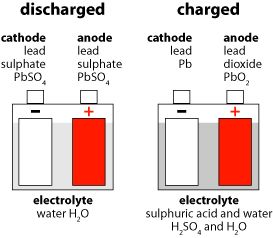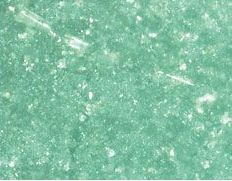# Batteries - How They work

A lead-acid battery cell consists of positive and negative lead plates of different composition suspended in a sulfuric acid solution called electrolyte. Electricity is the flow of electrons. When cells discharge, sulfur molecules from the electrolyte bond with the lead plates and releases electrons. When the cell recharges, excess electrons go back to the electrolyte. A battery develops voltage from this chemical reaction. In a typical lead-acid battery, the voltage is approximately 2 volts per cell regardless of cell size. Electricity flows from the battery as soon as there is a circuit between the positive and negative terminals. This happens when any load that needs electricity is connected to the battery.• Wattage, Volts, Amps, etc.

Most electrical appliances (in the US) are rated with wattage, a measure of energy consumption per unit of time. One watt delivered for one hour equals one watt-hour. Wattage is the product of current (amps) multiplied by voltage.
watt = amps x volt

One amp delivered at 120 volts is the same amount of wattage as 10 amps delivered 12 volts:
1 amp at 120 volts = 10 amps at 12 volts

Wattage is independent of voltage:
1 watt at 120 volts = 1 watt at 12 volts

To convert a battery's amp-hour capacity to watt-hours, multiply the amp-hours times the voltage.
The product is watt-hours.

To figure out how much battery capacity it will require to run a load (appliance) for a given time, multiply the appliance's wattage times the number of hours it will run to yield the total watt-hours. Then divide by the battery voltage to get the amp hours. For example, running a 60-watt load for one hour uses 60 watt-hours. If a 12-volt battery is supplying the load it will consume 5 amp-hours (60 watt-hours divided by 12 volts equals 5 amp-hours)

• Sulfation♦ Sulfation covering an electrode♦ A new electrode plate

Sulfation is the formation or deposit of lead sulfate (PbSO4) on the surface of the lead plates in the battery. If sulfation goes unchecked, and sits long enough the battery will loose efficiency or not work at all. Sulfation is commonly caused due to battery inactivity, operating in extreme temperatures, or prolonged over/under charging. Lead sulfate is created at both the positive and negative electrode plates during a discharge. In principle, during the charging period, 100% of the lead sulfate transforms to the positive plate (lead dioxide), the negative plate (lead) and sulfuric acid. However, in real life, when lead sulfate is left in the battery for a period of time, it crystallizes and becomes a hard sulfate that coats the surface of the electrode plates.

When hard lead sulfate coats the electrode plates, it causes a reduction in the area needed for the electro-chemical reactions and it also reduces the battery's available active material needed to maintain a high capacity.

The surface of a new electrode plate is spongy. This porous condition increases the surface area for the electro-chemical reaction. When an electrode plate is new and not coated by hard lead sulfate build-up, it has more area for electro-chemical reactions and more component materials to produce electricity.

Note:
Do not miss our FAQ on Lithium Battery Systems

 ↑ Recommend ZRD